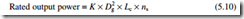# Generators:Rating and specification.

### Rating and specification

In order that a generator can be selected to suit a particular application, manufacturers issue specification data. This can be used and interpreted according to the following sections.

##### Rated output

The key aspect of the specification is the rated output of the generator, which is nor- mally expressed in terms of the apparent power (VA, kVA or MVA) when supplying the maximum load at the rated power factor, assumed to be 0.8. The rated output is usually based upon continuous operation in a maximum ambient temperature of 40°C. If the machine has a special short-time rating, the nameplate should state the time limits of operation.

The rated output from a given size of generator is related to the size and speed of the machine as follows:where Dg is the stator bore diameter, Lc is the stator core length and ns is the speed.

Here, K is a design constant which is proportional to the product of the airgap flux density and the stator current density.

The rated output of a machine is reduced in ambient temperatures exceeding 40°C and at altitudes above sea level exceeding 1000 m. The latter is because the air density is decreased, and its ability to cool the machine is reduced. Derating factors are applied for these conditions and typical values are summarized in Tables 5.1 and 5.2.

##### Reactances

The generator can be characterized by several reactances, each being useful in work- ing out the performance and protection requirements under different circumstances. These include the synchronous reactance, the transient reactance, the subtransient reactance, the Potier reactance and the negative- and zero-sequence reactances.

The subtransient reactance represents the output impedance of the generator within the first few cycles after a short-circuit occurs at the generator terminals. It is used for selecting protective relays for the connected load circuit. The lower the value of the subtransient reactance, the more onerous is the protection requirement.

Transient reactance represents the impedance of the machine over a slightly longer period, and is relevant to the performance of the generator and its AVR under changing load conditions. A low transient reactance is beneficial in responding to load changes.

Associated with the subtransient and transient reactances are time constants which define the rate of decay of these reactances.

The negative and positive sequence reactances influence the performance of the generator when supplying an unbalanced three-phase load.

##### Main items of specification

In summary, the following items are important when considering an application and specifying the appropriate generator:

● rated output, expressed as apparent power (VA)

● cooling air requirement (m3/s)

● moment of inertia of the rotor

● generator weight

● efficiency at full, 3/4, 1/2 and 1/4 load

● stator winding resistance

● reactances and time constants, as listed in section 5.7.2

● maximum short-circuit current delivered by the generator Typical values for a 200 kW generator are shown in Table 5.3.